# Inductors Tutorial

Inductors are coils of wire.

They may be wound on tubular FORMERS or may be self supporting.
The former may contain a metallic core up its centre.
Iron cores are used for frequencies below about 100 kHz.
Ferrite cores are used for frequencies up to say, 10 Mhz.
Above 100Mhz the core is usually air and the coil is self supporting.

At low frequencies the inductor may have hundreds of turns, above 1 Mhz only a few turns.

Most inductors have a low DC resistance since they are wound from copper wire.

Inductor values of INDUCTANCE are measured in HENRIES.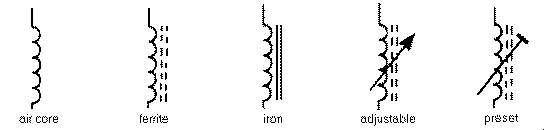Inductors oppose the flow of ac current.
This opposition is called INDUCTIVE REACTANCE.
Reactance increases with frequency and as the value of the inductance increases.

# Diode Tutorial

Diodes are polarised, which means that they must be inserted into the PCB the correct way round.
This is because an electric current will only flow through them in one direction (like air will only flow one way through a tyre valve).

Diodes have two connections, an anode and a cathode.
The cathode is always identified by a dot, ring or some other mark.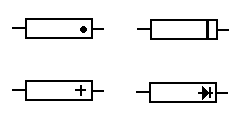The pcb is often marked with a + sign for the cathode end.

Diodes come in all shapes and sizes.
They are often marked with a type number.
Detailed characteristics of a diode can be found by looking up the type number in a data book.

If you know how to measure resistance with a meter then test some diodes. A good one has low resistance in one direction and high in the other.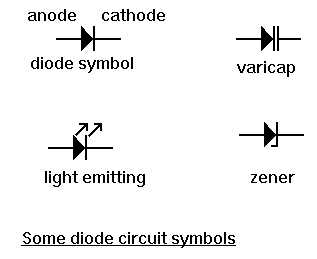There are specialised types of diode available such as the zener and light emitting diode (LED)

# Capacitors Tutorial

Capacitors are basically two parallel metal plates separated by an insulator.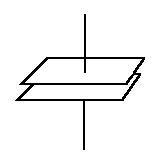This insulator is called the dielectric.
Capacitor types are named after the dielectric. Thus we have ceramic, mica, polyester, paper air capacitors etc.

Capacitors can be charged up and store electricity, similar to a car battery.
This can be a hazard if they are charged up to high voltages.
If it is necessary, capacitors with large charges should be discharged via a resistor to limit the discharge current.

DC current cannot flow through a capacitor since the dielectric forms an open circuit.

Capacitors come in all shapes and sizes and are usually marked with their value.
Values are measure in Farads. Values in Farads are unusual. Most capacitor values are measured in microfarads, nanofarads or picofarads. See the page on Value multipliers to find out more about this.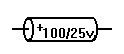They are often marked with their maximum working voltage. The voltage across the terminals must not exceed this value. It is OK to use a voltage below the maximum value.

Some capacitors such as electrolytic and tantalums are polarised. This means that they must be fitted the correct way round. They are marked to indicate polarity.

Some values are indicated with a colour code similar to resistors. There can be some confusion.
A 2200pf capacitor would have three red bands. These merge into one wide red band.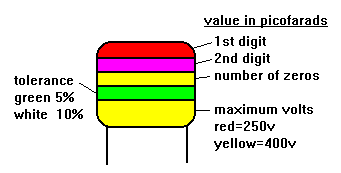Some values are marked in picofarads using three digit numbers. The first two digits are the base number and the third digit is a multiplier.
For example, 102 is 1000 pF and 104 is 100,000 pF = 100 nF = 0.1 uF.

To find the total value of capacitors in parallel (that is connected across each other) their values are added.
To find the total value if they are in series (that is in line with each other) then the following formula is used.

1/C total =1/C1 + 1/C2 + 1/C3 etc

Variable capacitors are available in which the value can be adjusted by controlling the amount of overlap of the plates or the distance between them.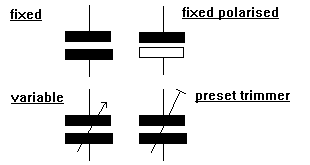There is a type of diode called the Varicap diode with similar characteristics.

Make a Free Website with Yola.